$\newcommand{\NN}{\mathbb{N}} \newcommand{\CC}{\mathbb{C}} \newcommand{\GG}{\mathbb{G}} \newcommand{\LL}{\mathbb{L}} \newcommand{\PP}{\mathbb{P}} \newcommand{\QQ}{\mathbb{Q}} \newcommand{\RR}{\mathbb{R}} \newcommand{\VV}{\mathbb{V}} \newcommand{\ZZ}{\mathbb{Z}} \newcommand{\FF}{\mathbb{F}} \newcommand{\KK}{\mathbb{K}} \newcommand{\UU}{\mathbb{U}} \newcommand{\EE}{\mathbb{E}} \newcommand{\Aa}{\mathcal{A}} \newcommand{\Bb}{\mathcal{B}} \newcommand{\Cc}{\mathcal{C}} \newcommand{\Dd}{\mathcal{D}} \newcommand{\Ee}{\mathcal{E}} \newcommand{\Ff}{\mathcal{F}} \newcommand{\Gg}{\mathcal{G}} \newcommand{\Hh}{\mathcal{H}} \newcommand{\Ii}{\mathcal{I}} \newcommand{\Jj}{\mathcal{J}} \newcommand{\Kk}{\mathcal{K}} \newcommand{\Ll}{\mathcal{L}} \newcommand{\Mm}{\mathcal{M}} \newcommand{\Nn}{\mathcal{N}} \newcommand{\Oo}{\mathcal{O}} \newcommand{\Pp}{\mathcal{P}} \newcommand{\Qq}{\mathcal{Q}} \newcommand{\Rr}{\mathcal{R}} \newcommand{\Ss}{\mathcal{S}} \newcommand{\Tt}{\mathcal{T}} \newcommand{\Uu}{\mathcal{U}} \newcommand{\Vv}{\mathcal{V}} \newcommand{\Ww}{\mathcal{W}} \newcommand{\Xx}{\mathcal{X}} \newcommand{\Yy}{\mathcal{Y}} \newcommand{\Zz}{\mathcal{Z}} \newcommand{\al}{\alpha} \newcommand{\la}{\lambda} \newcommand{\ga}{\gamma} \newcommand{\Ga}{\Gamma} \newcommand{\La}{\Lambda} \newcommand{\Si}{\Sigma} \newcommand{\si}{\sigma} \newcommand{\be}{\beta} \newcommand{\de}{\delta} \newcommand{\De}{\Delta} \renewcommand{\phi}{\varphi} \renewcommand{\th}{\theta} \newcommand{\om}{\omega} \newcommand{\Om}{\Omega} \renewcommand{\epsilon}{\varepsilon} \newcommand{\Calpha}{\mathrm{C}^\al} \newcommand{\Cbeta}{\mathrm{C}^\be} \newcommand{\Cal}{\text{C}^\al} \newcommand{\Cdeux}{\text{C}^{2}} \newcommand{\Cun}{\text{C}^{1}} \newcommand{\Calt}{\text{C}^{#1}} \newcommand{\lun}{\ell^1} \newcommand{\ldeux}{\ell^2} \newcommand{\linf}{\ell^\infty} \newcommand{\ldeuxj}{{\ldeux_j}} \newcommand{\Lun}{\text{\upshape L}^1} \newcommand{\Ldeux}{\text{\upshape L}^2} \newcommand{\Lp}{\text{\upshape L}^p} \newcommand{\Lq}{\text{\upshape L}^q} \newcommand{\Linf}{\text{\upshape L}^\infty} \newcommand{\lzero}{\ell^0} \newcommand{\lp}{\ell^p} \renewcommand{\d}{\ins{d}} \newcommand{\Grad}{\text{Grad}} \newcommand{\grad}{\text{grad}} \renewcommand{\div}{\text{div}} \newcommand{\diag}{\text{diag}} \newcommand{\pd}{ \frac{ \partial #1}{\partial #2} } \newcommand{\pdd}{ \frac{ \partial^2 #1}{\partial #2^2} } \newcommand{\dotp}{\langle #1,\,#2\rangle} \newcommand{\norm}{|\!| #1 |\!|} \newcommand{\normi}{\norm{#1}_{\infty}} \newcommand{\normu}{\norm{#1}_{1}} \newcommand{\normz}{\norm{#1}_{0}} \newcommand{\abs}{\vert #1 \vert} \newcommand{\argmin}{\text{argmin}} \newcommand{\argmax}{\text{argmax}} \newcommand{\uargmin}{\underset{#1}{\argmin}\;} \newcommand{\uargmax}{\underset{#1}{\argmax}\;} \newcommand{\umin}{\underset{#1}{\min}\;} \newcommand{\umax}{\underset{#1}{\max}\;} \newcommand{\pa}{\left( #1 \right)} \newcommand{\choice}{ \left\{ \begin{array}{l} #1 \end{array} \right. } \newcommand{\enscond}{ \left\{ #1 \;:\; #2 \right\} } \newcommand{\qandq}{ \quad \text{and} \quad } \newcommand{\qqandqq}{ \qquad \text{and} \qquad } \newcommand{\qifq}{ \quad \text{if} \quad } \newcommand{\qqifqq}{ \qquad \text{if} \qquad } \newcommand{\qwhereq}{ \quad \text{where} \quad } \newcommand{\qqwhereqq}{ \qquad \text{where} \qquad } \newcommand{\qwithq}{ \quad \text{with} \quad } \newcommand{\qqwithqq}{ \qquad \text{with} \qquad } \newcommand{\qforq}{ \quad \text{for} \quad } \newcommand{\qqforqq}{ \qquad \text{for} \qquad } \newcommand{\qqsinceqq}{ \qquad \text{since} \qquad } \newcommand{\qsinceq}{ \quad \text{since} \quad } \newcommand{\qarrq}{\quad\Longrightarrow\quad} \newcommand{\qqarrqq}{\quad\Longrightarrow\quad} \newcommand{\qiffq}{\quad\Longleftrightarrow\quad} \newcommand{\qqiffqq}{\qquad\Longleftrightarrow\qquad} \newcommand{\qsubjq}{ \quad \text{subject to} \quad } \newcommand{\qqsubjqq}{ \qquad \text{subject to} \qquad }$

Sparse Spikes Deconvolution with MUSIC Algorithm

# Sparse Spikes Deconvolution with MUSIC Algorithm

This numerical tour explores the use of the MUSIC algorithm to perform sparse deconvolution.

## Contents

The MUSIC algorithm was introduced by [Schmidt]. It is closely related to Prony's method [Prony], and is very popular in signal processing [KrimViberg], where it is mostly used for line spectral estimation (i.e. find locations of Diracs in the Fourier spectrum), and we re-formulate here as a problem of finding Diracs' over the temporal domain.

We follow here the exposition of [LiaoFannjiang] who propose a theoretical analysis of the performances of this method. Several related algorithms exists, see for instance [RoyKailathHuaSarkar,DemNeedNg].

## Installing toolboxes and setting up the path.

You need to download the following files: signal toolbox and general toolbox.

You need to unzip these toolboxes in your working directory, so that you have toolbox_signal and toolbox_general in your directory.

For Scilab user: you must replace the Matlab comment '%' by its Scilab counterpart '//'.

Recommandation: You should create a text file named for instance numericaltour.sce (in Scilab) or numericaltour.m (in Matlab) to write all the Scilab/Matlab command you want to execute. Then, simply run exec('numericaltour.sce'); (in Scilab) or numericaltour; (in Matlab) to run the commands.

Execute this line only if you are using Matlab.

getd = @(p)path(p,path); % scilab users must *not* execute this


Then you can add the toolboxes to the path.

getd('toolbox_signal/');
getd('toolbox_general/');
ms = 20; % markersize


## MUSIC Algorithm

We consider here the problem of recovering a Radon measure $$m_0 \in \Mm(\mathbb{T})$$ defined on the torus $$\mathbb{T}=\RR/\ZZ$$ from of possibly noisy low-pass measurements $y = y_0 + w \qwhereq y_0 = \Phi m_0$ where $$w$$ is the noise term, and so that $$\Phi m$$ computes the first $$M+1$$ low frequencies of the Fourier transform of $$m$$, i.e. $\forall \ell \in \{0,\ldots,M\}, \quad (\Phi m)_\ell = \int_{\mathbb{T}} e^{-2\imath\pi x \ell} d m(x).$ We only consider positive frequency because we assume $$m$$ is a real measure.

In the following, we consider a discrete measure of the form $$m_0=m_{a_0,x_0}$$ where we used the following notation $m_{a,x} = \sum_{i=1}^N a_i \de_{x_i}$ where $$a \in \RR^N$$ and $$x \in \mathbb{T}^N$$.

We thus have $$\Phi m_{a,x} = \Phi_x^M a$$ where $\Phi_x^{M} = ( e^{-2\imath\pi x_j \ell} )_{0 \leq\ell \leq M, 1 \leq j \leq N} \in \RR^{(M+1) \times N}.$

Note that $$\Phi_x^{M}$$ is a (rectangular) Vandermonde matrix, since $\Phi_x^M = (V_{\ga(x)}^M)^* \qwhereq \ga(x) = ( e^{2\imath\pi x_j} )_{j=1}^N \in \CC^N.$

For $$y \in \RR^M$$ and for $$0<L<M$$ we denote the Hankel matrix $H_y = \begin{pmatrix} y_0 & y_1 & \ldots & y_{M-L} \\ y_1 & y_2 & \ldots & y_{M-L+1} \\ \vdots & \vdots & \ddots & \vdots \\ y_L & y_{L+1} & \ldots & y_M \end{pmatrix} \in \RR^{(L+1) \times (M-L+1) }.$

Since $$y=\Phi_x^M a+w$$, one can check that one has the following factorization $H_y = \Phi_x^{L} \diag(a) ( \Phi_x^{M-L} )^* + H_{w}.$

We suppose that $$\min(L,M-L+1) \geq N$$. The fundamental property, which is based on the above factorization, is that $s \in \{x_{0,1},\ldots,x_{0,N}\} \quad\Longleftrightarrow\quad \phi_L(s) \in \text{Im}(H_{y_0}) \qwhereq \phi_L(s) = (e^{-2\imath\pi \ell s })_{0 \leq s \leq L} \in \CC^{L+1}.$

From observations $$y$$, we define the following detection function $\forall s \in \mathbb{T}, \quad d_y(s) = \norm{ U^{\bot,*} \phi^L(s) }^2,$ where we considered the following SVD factorization $H_y = [U, U^\bot] \diag_j(\si_j) [V, V^\bot]^*$ $\qwhereq \choice{ U \in \CC^{(L+1) \times N}, U^\bot \in \CC^{(L+1) \times (L+1-N)} \\ V \in \CC^{(M-L+1) \times N}, V^\bot \in \CC^{(M-L+1) \times (M-L+1-N)}. }$

The detection property can hence be conveniently re-written using this detection function as $\{x_{0,1},\ldots,x_{0,N}\} = \enscond{ s }{ d_{y_0}(s)=0 }.$

## Sparse Spikes Deconvolution

We compute here some low frequency measurements.

Number $$M$$ of recorded frequency.

M = 18;


Imaging grid, for display purpose.

Q = 1024*4;
z = (0:Q)'/Q;


Input positions $$x_0 \in \mathbb{T}^N$$.

x0 = [.1 .4 .5 .7 .9]';


Number $$N$$ of spikes.

N = 5;


Input amplitudes $$a_0 \in \RR^N$$.

a0 = [.7 -.8 .9 1 -.9]';


Observation operator at position $$x$$, i.e. $$\Phi_x$$.

Phi = @(x)exp(-2i*pi*(0:M)'*x(:)');


Observations $$y=y_0+w$$.

sigma = .15;
w = (randn(M+1,1)+1i*randn(M+1,1)); w = w/norm(w);
y0 = Phi(x0)*a0;
y = y0 + sigma*norm(y0)*w;


Compute the observed low frequency signal $f = \frac{1}{2M+1} \sum_{\ell=-P}^P y_\ell e^{2\imath\pi \ell \cdot}$ where we completed the observation using $$y_{-\ell} = \bar y_{\ell}$$ since the input measure is real.

PhiT = @(x)exp(2i*pi*x(:)*(-M:M)) / (2*M+1);
f = real( PhiT(z) * [conj(y(end:-1:2)); y]);


Display the observed signal $$f$$, together with the spikes locations.

clf; hold on;
plot(z, f);
stem(x0,a0, 'r');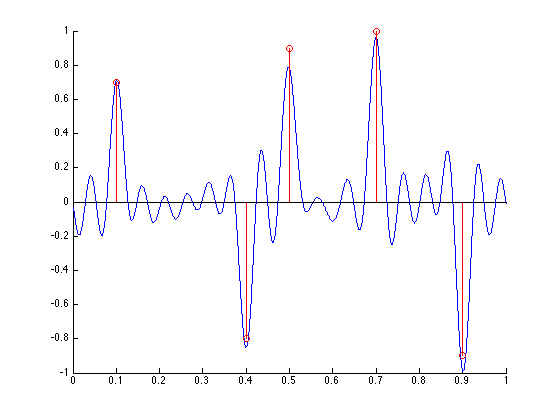## Noiseless Recovery

We first check the exact recovery when using $$y_0$$.

We set here $$L=M/2$$, which is a standard choice.

L = M/2;


Build Hankel matrix, one must have min(size(H))>N.

MusicHankel = @(y)hankel(y(1:L),y(L:M));


Compute SVD of $$H_{y_0}$$.

[U,S,V] = svd(MusicHankel(y0),0);
S = diag(S);


Display the decay of the eigenvalues of $$H_{y_0}$$. Since there is no noise, only the $$N$$ largest one are non-zero. This is a convenient way to detect $$N$$ if it is unknown.

clf;
plot(S, '.-', 'MarkerSize', ms);
axis tight;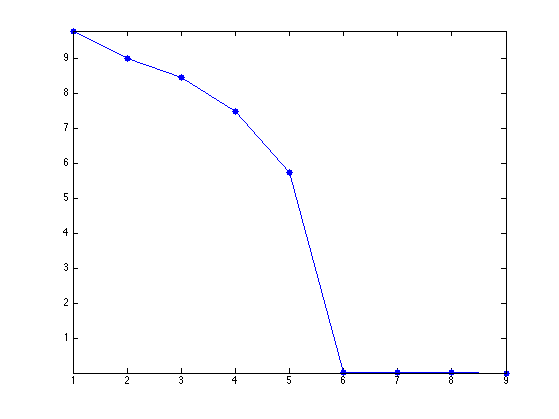Imaging function $$d_{y_0}$$, evaluated on a thin grid $$z \in \mathbb{T}^Q$$.

Ubot = U(:,N+1:end);
d = Ubot'*exp(-2i*pi*(0:L-1)'*z(:)');
d = sum(abs(d).^2) / L;


Display $$d$$.

clf; hold on;
plot(z, d, 'b');
stem(x0, 1+x0*0, 'r.');
axis([0 1 0 1]); box on;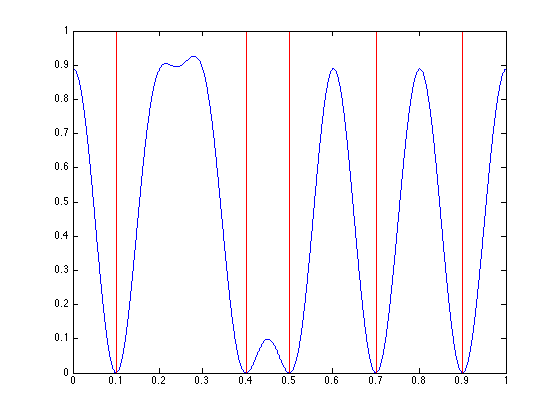## Computing the Diracs' Locations by Root Finding

Instead of evaluating the function $$d_{y_0}$$ on a thin grid, it is possible to compute directly its zeros using root finding.

Indeed, one has $d_y(s) = \norm{ U^{\bot,*} \phi^L(s) }^2 = \sum_j \sum_{\ell,\ell'} \bar U^{\bot}_{\ell,j} U^{\bot}_{\ell',j} z^{\ell-\ell'}$ where we denoted $$z=e^{2\imath\pi s}$$.

So the zeros of $$d_y$$ are equal to the zeros of the polynomial $$P_y$$ that are on the unit circle, where we introduced $P_y(z) = \sum_\ell C_\ell z^\ell \qwhereq \choice{ C_\ell = \sum_{j} B_{j,\ell}, \\ B_{j,\cdot} = U_{\cdot,j} \star \bar U_{-\cdot,j}, \\ }$ where $$\star$$ is the product of convolution.

This means that $\enscond{s}{d_y(s)=0} = \frac{1}{2\pi} \text{Angle}\pa{ \enscond{z}{P_y(z)=0 \qandq \abs{z}=1} }$ where Angle returns the angle of a complex number.

Compute the coefficients $$C$$ of the polynomial $$P_{y_0}$$.

B = [];
for j=1:size(Ubot,2)
u = Ubot(:,j);
v = flipud(conj(u));
B(:,j) = conv(u,v);
end
C = sum(B,2);


Find its roots.

R = roots(C(end:-1:1));


Locate those that are along the circle.

R1 = R(abs( abs(R)-1 )<1e-4);


Display roots.

clf; hold on;
plot(exp(2i*pi*z), 'k');
plot(R, 'b.', 'MarkerSize', ms);
plot(R1, 'r.', 'MarkerSize', ms);
axis equal; box on;
axis([-1 1 -1 1]*2);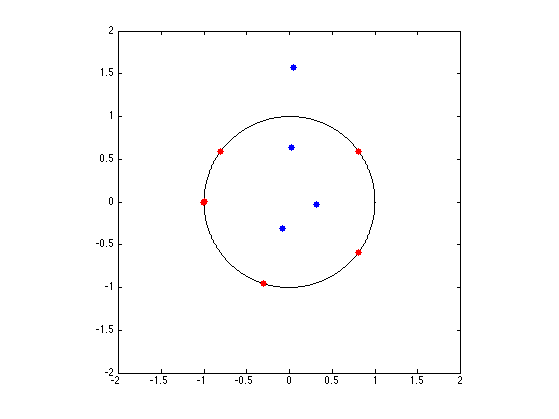Sort according to angle, and discard one out of two (because of double roots).

[x1,I] = sort(mod(angle(R1),2*pi)/(2*pi));
x1 = x1(1:2:end);


Compare the found locations with the ground trust.

disp( ['Error (should be 0): ' num2str(norm(x1-x0))] );

Error (should be 0): 3.1113e-09


## Noisy Recovery

In practice, one has access to noisy observation $$y=y_0+w$$, and one approximates the support $$x$$ using the best $$N$$ local minima $$\tilde x = \{\tilde x_1,\ldots,\tilde x_N\} \in \mathbb{T}^N$$ of $$d_{y}$$.

Exercice 1: (check the solution) Compute and display $$d_y$$

exo1;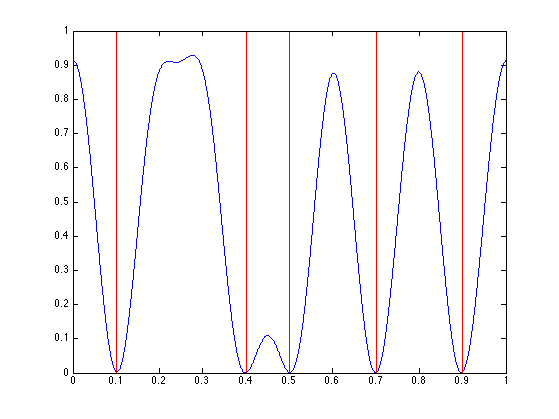Exercice 2: (check the solution) Display the roots of $$P_y$$ that are inside the unit disk

exo2;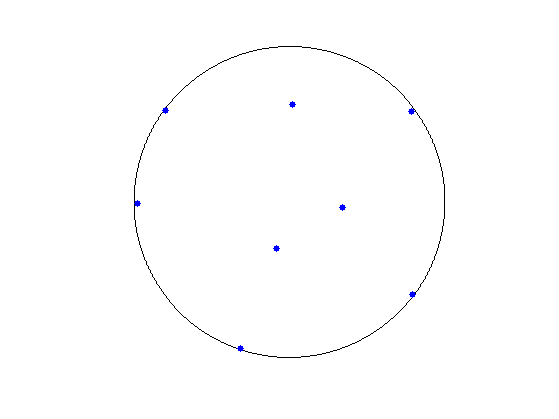Exercice 3: (check the solution) Keep only the best $$N$$ ones, i.e. those that are the closest from the unit circle. We denote those as $$\tilde x \in \mathbb{T}^N$$. Recover an approximation $$\tilde a$$ of the amplitudes $$a_0$$ By solving in least squares (using backslash operator) the system $$\Phi_{\tilde x} \tilde a = y.$$ Display the recovered measure $$m_{\tilde a, \tilde x}$$.

exo3;Exercice 4: (check the solution) Display the evolution of roots as the noise level $$\sigma$$ increases.

exo4;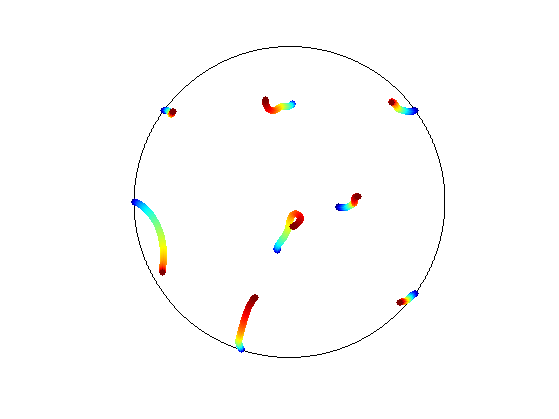## Bibliography

• [Schmidt] R. Schmidt, Multiple emitter location and signal parameter estimation, IEEE Trans. on Antennas and Propagation, vol. 34, no. 3, pp. 276-280, 1986.
• [LiaoFannjiang14] W. Liao, A. Fannjiang, MUSIC for Single-Snapshot Spectral Estimation: Stability and Super-resolution, 2014.
• [Prony] G. R. B. de Prony, Essai Experimentale et Analytique, J. de L'Ecole Polytechnique 2, pp. 24-76, 1795.
• [KrimViberg] H. Krim and M. Viberg, Two decades of array signal processing research: the parametric approach, IEEE Signal Processing Magazine 13(4), pp.67-94, 1996.
• [RoyKailath] R. Roy and T. Kailath, ESPRIT ? Estimation of signal parameters via rotation invariance techniques, IEEE Trans. Acoust., Speech, Signal Proc., vol. 17, no. 7, July 1989
• [HuaSarkar] Y. Hua and T. K. Sarkar, Matrix pencil method for estimating parameters of exponentially damped/undamped sinusoids in noise, IEEE Transactions on Acoustics, Speech and Signal Processing 38(5), pp.814-824, 1990.
• [DemNeedNg] L. Demanet, D. Needell and N. Nguyen, Super-resolution via superset selection and pruning, Proceedings of the 10th International Conference on Sampling Theory and Applications, 2013.